Calculating Spectral Weights for mkinstmapOverview

Synopsis:

When a spectral weights file is provided to mkinstmap, the tool computes a weighted instrument map instead of a monochromatic instrument map. Essentially, a weighted instrument map is a linear combination of monochromatic maps calculated for several energy-weight pairs. More details are given in the Why are weighted instrument maps important? section of this thread.

Purpose:

Use the make_instmap_weights script or Sherpa to create a spectral weights file that can be used by mkinstmap to create a weighted instrument map for use in one of the exposure map threads.

Last Update: 22 Dec 2014 - Review for CIAO 4.7; no changes.

Get Started

No data file is actually needed to create the weights file. As we create instrument- and exposure- maps to see what difference adding a weights file makes, we require the same data products for these steps as the Single Chip Exposure Map thread.

Why are weighted instrument maps important?

In dividing a counts image by an exposure map, the primary aim is to obtain an image with units of surface brightness in photons/s/cm2/arcsec2 or, equivalently, in flux/pixel. It is important to understand that this can yield an accurate answer only if the number of counts in each pixel is actually proportional to the integral of the incident source spectrum over the relevant energy band.

Due to the strong energy dependence of the effective area, this is not always true. In particular, the number of detected counts is proportional to the incident flux in a broad band only when the effective area is constant within the chosen band. Large systematic errors may result if one blindly applies a monochromatic exposure map to a broad band image which encompasses a large range of variation in the effective area.

Nevertheless, it is often desirable both to examine broader energy bands and to examine energy bands where the response is not particularly flat. In such cases, if the broad features of the incident spectrum are known (e.g. thermal emission from a cluster) and if the spectral variations within the image are not too large, one can reduce the systematic errors by computing an exposure map which is weighted according to a specific model for the incident spectrum. An Introduction to Exposure Maps (PS, 12pp) gives a comparison of MARX simulated data analyzed using both weighted and monochromatic exposure maps.

Using the make_instmap_weights script

The make_instmap_weights script takes a Sherpa model and an optional energy range and produces a weighted spectrum file.

For this example we are going to use a power law, with a gamma of 1.7, which is absorbed by a line-of-sight column density of 1.3 x 1022 atoms cm-2. The models and parameter values should be changed to match the emission from the source of interest. The model is evaluated over the energy range of 0.5-8.0 keV with bins that are 0.25 keV wide:

```unix% punlearn make_instmap_weights
unix% make_instmap_weights weights.txt "xsphabs.gal*powlaw1d.p1" \
paramvals="gal.nh=1.3;p1.gamma=1.7" emin=0.5 emax=8 ewidth=0.25
Model used:
(xsphabs.gal * powlaw1d.p1)
Param        Type          Value          Min          Max      Units
-----        ----          -----          ---          ---      -----
gal.nH       thawed          1.3            0       100000 10^22 atoms / cm^2
p1.gamma     thawed          1.7          -10           10
p1.ref       frozen            1 -3.40282e+38  3.40282e+38
p1.ampl      thawed            1            0  3.40282e+38
Created: weights.txt
```

The output file, weights.txt, contains the energy bin center in keV in the first column and normalized weighting in the second column:

```unix% cat weights.txt
#TEXT/SIMPLE
# X WEIGHT
#
# DATE = 2013-12-02T18:46:56 / Date and time of file creation
# MODELEXPR = (xsphabs.gal * powlaw1d.p1)
# ENERG_LO = 0.5 / [keV] Minimum energy
# ENERG_HI = 8.0 / [keV] Maximum energy
#
0.625 0.000262206958899
0.875 0.00680336709152
1.125 0.0296492945584
1.375 0.0562062174781
1.625 0.0705678030635
1.875 0.0740071023859
2.125 0.0706968736338
2.375 0.06683637039
2.625 0.0605703912684
2.875 0.0554519470171
3.125 0.0504631354041
3.375 0.0457218204413
3.625 0.0416093446645
3.875 0.0379342170434
4.125 0.0346277236647
4.375 0.0317287277273
4.625 0.029175094479
4.875 0.0269070452828
5.125 0.0248881325335
5.375 0.0230860958395
5.625 0.0214728313009
5.875 0.0200240167049
6.125 0.0187147444077
6.375 0.0175361040711
6.625 0.0164677939141
6.875 0.0154966568871
7.125 0.0145206381483
7.375 0.0135493374534
7.625 0.0128396753267
7.875 0.0121852908602
```

To set the file for use in the spectrumfile parameter of mkinstmap:

```unix% pset mkinstmap spectrumfile=weights.txt
```

Step-by-Step Guide

This section explains how to manually run the analysis steps and examine the changes that are made with different weight files.

This section of the thread uses the sherpa_contrib utilities package, which can be loaded into Sherpa using the following command:

```sherpa> from sherpa_contrib.utils import *
```

Summary of the Syntax

An example set of commands used to create the weight file follows; please remember to change the data range, binning, spectral model and parameters to those appropriate for your data!

```sherpa> from sherpa_contrib.utils import *
sherpa> dataspace1d(0.5, 8, 0.25)
sherpa> set_source(xsphabs.gal * powlaw1d.pl)
sherpa> gal.nh = 1.3
sherpa> pl.gamma = 1.7
sherpa> save_instmap_weights("weights.txt")
Created: weights.txt
```

The output file - here called weights.txt - can then be used as the input for the spectrumfile parameter of mkinstmap.

Create a model in Sherpa

Since the weights are largely independent of the responses, it is not necessary to use the PHA and ARF files to define a model. Instead, we can use the Sherpa dataspace1d command to create an energy grid from 0.5 to 8 keV with a bin width of 0.25 keV:

```sherpa> dataspace1d(0.5, 8, 0.25)
```

Now we want to define a model that represents the source emission: we do not need to worry about the overall normalization of the model, just that the relative amplitudes of any emission components is correct.

For this example we are going to use a power law, with a gamma of 1.7, which is absorbed by a line-of-sight column density of 1.3 x 1022 atoms cm-2. The models and parameter values should be changed to match the emission from the source of interest.

```sherpa> set_source(xsphabs.gal * powlaw1d.pl)
sherpa> gal.nh = 1.3
sherpa> pl.gamma = 1.7
sherpa> plot_source()
sherpa> log_scale()
```

The plot of the spectrum is shown in Figure 1:

Figure 1: Source spectrum[Version: PNG, postscript, PDF]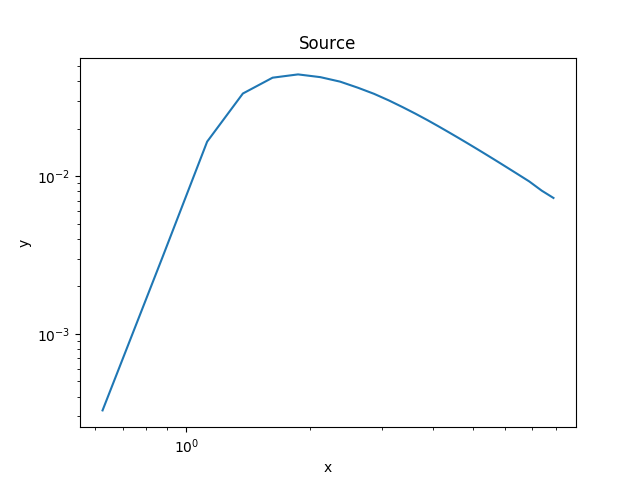Figure 1: Source spectrum

The source model evaluated on the energy grid that will be used to create the weights. The normalisation of the powerlaw model is not important here, since the calculation of the weights involves normalizing the model values by the total model flux.

Plot the weights

The plot_instmap_weights routine plots up the weights, as shown in Figure 2.

```sherpa> plot_instmap_weights()
sherpa> log_scale()
```

Figure 2: Weights using a bin width of 0.25 keV[Version: PNG, postscript, PDF]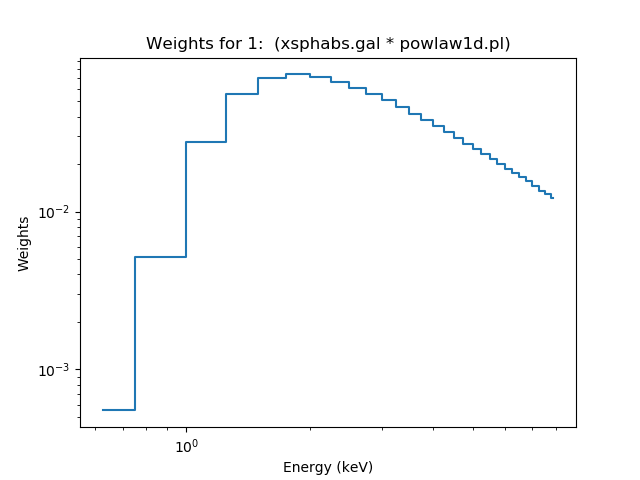Figure 2: Weights using a bin width of 0.25 keV

Since we are evaluating the weights on the same grid used to calculate the source spectrum (Figure 1), the shape of the two plots are the same; the only differences are the magnitude of the Y axis and that the data is now plotted as a histogram.

Save the weights

The weights can be saved to an ASCII file, in a format suitable for mkinstmap using the save_instmap_weights routine. Here we save them to the file "weights.txt".

```sherpa> save_instmap_weights("weights.txt")
Created: weights.txt
```

Since the file is in ASCII format you can view it with various UNIX utilities, or with the dmlist and prism tools:

```unix% head -10 weights.txt
#TEXT/SIMPLE
# X WEIGHT
#
# DATE = 2013-12-02T18:49:48 / Date and time of file creation
# MODELEXPR = (xsphabs.gal * powlaw1d.pl)
# ENERG_LO = 0.5 / [keV] Minimum energy
# ENERG_HI = 8.0 / [keV] Maximum energy
#
0.625 0.000262206958899
0.875 0.00680336709152
unix% tail -5 weights.txt
6.875 0.0154966568871
7.125 0.0145206381483
7.375 0.0135493374534
7.625 0.0128396753267
7.875 0.0121852908602
```

The dmstat tool can be used to show that the weights column sums up to 1, as is needed for use in mkinstmap:

```unix% dmstat weights.txt
X
min:	0.625 	      @:	1
max:	7.875 	      @:	30
mean:	4.25
sigma:	2.1638603621
sum:	127.5
good:	30
null:	0

WEIGHT
min:	0.0002622069589 	      @:	1
max:	0.074007102386 	      @:	6
mean:	0.033333333333
sigma:	0.020733677725
sum:	1
good:	30
null:	0
```

The output table gives the energy at the center of each band (in keV, X) and the fraction of the incident flux falling in that band (the spectral weighting, WEIGHT). This file may now be used to create a weighted instrument map; e.g. see the Compute an ACIS Exposure Map (Single Chip) and Build Fluxed Image thread.

In the following sections of the thread we show how you can change the binning used to create the weights and visualize the differences. Note that using a bin width of 0.25 keV is generally excessive, in that it over-resolves the energy grid, and so will lead to longer run times of mkinstmap than are strictly necessary.

Comparing weights

If you wish to use a different energy range then you can call dataspace1d to create a new grid, or filter the grid by using the ignore and notice routines. In the following we create new datasets with different binning characteristics to investigate the differences these choices can make.

First we use the same energy range but double the bin width to 0.5 keV. We add the weights to the existing plot (Figure 2) rather than create a new plot, and so create Figure 3:

```sherpa> dataspace1d(0.5, 8, 0.5, id=2)
sherpa> set_source(2, get_source())
sherpa> plot_instmap_weights(2, overplot=True)
sherpa> set_histogram(["line.color", "red"])
sherpa> save_instmap_weights(2, "weights2.txt")
Created: weights2.txt
```

Figure 3: A comparison of bin widths of 0.25 and 0.5 keV[Version: PNG, postscript, PDF]Figure 3: A comparison of bin widths of 0.25 and 0.5 keV

The red line, from the second set of weights, is roughly twice as high as the original plot. The actual difference depends on how much the model varies within the new bins.

We now decide to use variable-width bins, manually choosing energy ranges over which we consider the effective area to be approximately flat. These values are for demonstration purposes only and should not be considered to be valid choices for your analysis!

As the dataspace1d command only creates regularly-spaced grids, we use the load_arrays command to create the grid: the xlo*0 statement is used to create a fake data array, since we ignore the data values for this calculation. The result is shown in Figure 4.

```sherpa> edges = [0.5, 1, 1.5, 2, 2.25, 2.5, 2.75, 3, 3.5, 4, 6, 8]
sherpa> xe = numpy.asarray(edges)
sherpa> xlo = xe[:-1]
sherpa> xhi = xe[1:]
sherpa> load_arrays(3, xlo, xhi, xlo*0, Data1DInt)
sherpa> set_source(3, get_source())
sherpa> plot_instmap_weights(3, overplot=True)
sherpa> set_histogram(["line.color", "green"])
sherpa> save_instmap_weights(3, "weights3.txt")
Created: weights3.txt
```

Figure 4: Using a variable bin width[Version: PNG, postscript, PDF]Figure 4: Using a variable bin width

The green line, showing the weights from the variable bin widths, is similar to the second (red) set of weights since the bin widths are not too different.

It may be useful to plot the ARF for your source along with the weights, to check that the binning you have used is sensible. In the following we use ChIPS commands to plot the data from the file src.arf on top of the weights, creating Figure 5:

```sherpa> plot_instmap_weights(3)
sherpa> set_plot_ylabel("ARF (cm^2)")
sherpa> log_scale(Y_AXIS)
sherpa> limits(Y_AXIS, 30, AUTO)
sherpa> limits(X_AXIS, 0, 9)
```

Figure 5: Comparing a set of weights to an ARF[Version: PNG, postscript, PDF]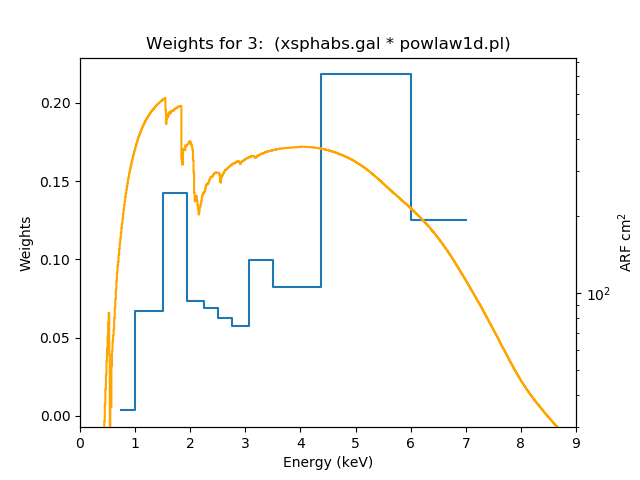Figure 5: Comparing a set of weights to an ARF

By adding a second Y axis to the plot, the ARF can be shown in the same plot, even though the Y axis values for the two data sets are very different.

The plot can be used to help you determine whether the choice of bins is sensible or not by seeing how the ARF varies within a bin. Ideally, the bins should be chosen to define regions where the effective area curve is roughly flat (slowly varying with energy); the reasons for this are discussed in An Introduction to Exposure Maps (PS, 12pp).

How do the resulting instrument maps differ?

In this section we create instrument maps for the weights created above, and compare them to a mono-energetic version. All the instrument maps were created using the following set of parameters (taken from the single chip exposure map thread):

```unix% punlearn mkinstmap
unix% pset mkinstmap pixelgrid="1:1024:#1024,1:1024:#1024"
unix% pset mkinstmap obsfile=repro/acisf01838_repro_evt2.fits
unix% pset mkinstmap detsubsys=ACIS-7
```In this example, since we are only interested in how the effective area varies with the weighting, we have not used acis_set_ardlib to ensure that the observation-specific bad-pixel file has been used.

The maps that were created are given below:

Mono-energetic: imap.e1.7.fits
```unix% mkinstmap imap.e1.7.fits NONE 1.7 mode=h
```
0.25 keV bin width: imap.weights.fits
```unix% mkinstmap imap.weights.fits weights.txt 1 mode=h
```
0.5 keV bin width: imap.weights2.fits
```unix% mkinstmap imap.weights2.fits weights2.txt 1 mode=h
```
Variable bin width: imap.weights3.fits
```unix% mkinstmap imap.weights3.fits weights3.txt 1 mode=h
```

On a reasonably modern Linux machine the run times for mkinstmap were approximately 5 s, 20 s, 10 s, and 10 s for these four maps (rounded to the nearest 5 seconds). The actual run times depend strongly on operating system and disk access, so these numbers should only be taken as rough guides.

A quick comparison using dmstat shows that the three weighted maps are similar, but significantly different to the mono-energetic case:

```unix% dmstat imap\*fits cen- med+ sig-
File=imap.e1.7.fits
IMAP[cm**2]
min:	0 	      @:	( 1 1 )
max:	634.50579834 	      @:	( 212 432 )
mean:	594.61666063
median:	603.82928467
sum:	623500759.54
good:	1048576
null:	0
File=imap.weights.fits
IMAP[cm**2]
min:	0 	      @:	( 1 1 )
max:	416.92279053 	      @:	( 212 425 )
mean:	384.12750188
median:	390.36341858
sum:	402786879.41
good:	1048576
null:	0
File=imap.weights2.fits
IMAP[cm**2]
min:	0 	      @:	( 1 1 )
max:	417.46255493 	      @:	( 212 425 )
mean:	384.83357629
median:	391.03649902
sum:	403527252.09
good:	1048576
null:	0
File=imap.weights3.fits
IMAP[cm**2]
min:	0 	      @:	( 1 1 )
max:	416.90100098 	      @:	( 212 425 )
mean:	383.78799585
median:	389.97721863
sum:	402430881.54
good:	1048576
null:	0
```

Comparing the median values - shown in bold above - shows that the weighted maps are roughly 65 % of the mono-energetic value. Dividing imap.weights.fits by imap.e1.7.fits produces the image shown in Figure 6.

```unix% punlearn dmimgcalc
unix% dmimgcalc imap.weights.fits,imap.e1.7.fits out=ratio1.fits op=imgout=img1/img2 mode=h
unix% dmstat ratio1.fits cen- sig- med+
ratio1.fits
min:	0.60734897852 	      @:	( 594 624 )
max:	0.65879666805 	      @:	( 220 2 )
mean:	0.64585134846
median:	0.64655774832
sum:	667818.69038
good:	1034013
null:	14563
```

The use of imgout=img1/img2 for the operation parameter of dmimgcalc ensures that 0-pixel values get replaced by NaN values, and so will be ignored by dmstat, ChIPS, and ds9. If we had used op=div and set both infile and infile2 then these pixels would have been set to 0 instead.

```unix% chips -n
chips> reposition_plot(0, 0, 1, 1)
chips> set_plot(['style', 'open'])
chips> add_colorbar(0.4, 0.9, ["width", 0.05, "length", 0.6])
chips> set_colorbar(["*.color", "black", "*.thickness", 2,
"ticklabel.size", 16, "ticklabel.fontstyle", "bold"])
```

Figure 6: Ratio of a weighted instrument map to a mono-energetic one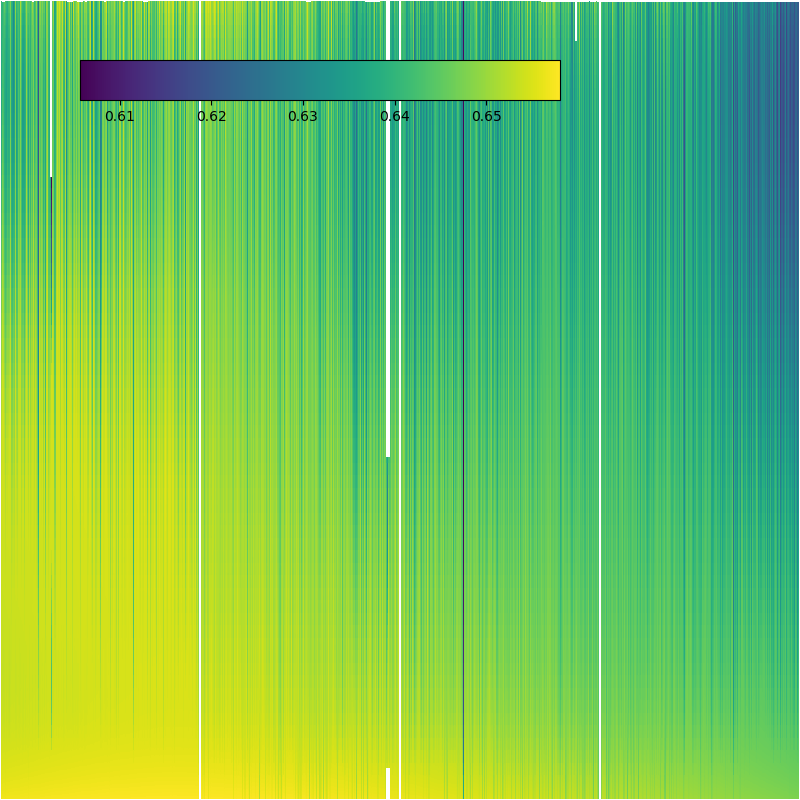Figure 6: Ratio of a weighted instrument map to a mono-energetic one

The ratio image shows that whilst there is a lot of small-scale differences - the ACIS-S instrument maps are calibrated per column and there are horizontal and vertical gradients - the overall image is flat within a 5% margin. The large-scale variations are more relevant since the small-scale structure tends to get washed out when creating an exposure map due to the Chandra dither pattern (see Figure 9).

In general the per-column variation is due to the variation in the detector response (the Quantum Efficiency), whilst the large-scale differences are due to the off-axis dependence of the effective area.

Comparing the weighted instrument maps show that the absolute variation between them is much smaller than to the mono-energetic case (Figure 7 and Figure 8):

```unix% dmimgcalc imap.weights2.fits,imap.weights.fits out=ratio2.fits op=imgout=img1/img2 mode=h
unix% dmimgcalc imap.weights3.fits,imap.weights.fits out=ratio3.fits op=imgout=img1/img2 mode=h
```

Figure 7: Ratio of 0.5 keV to 0.25 keV bin-width instrument maps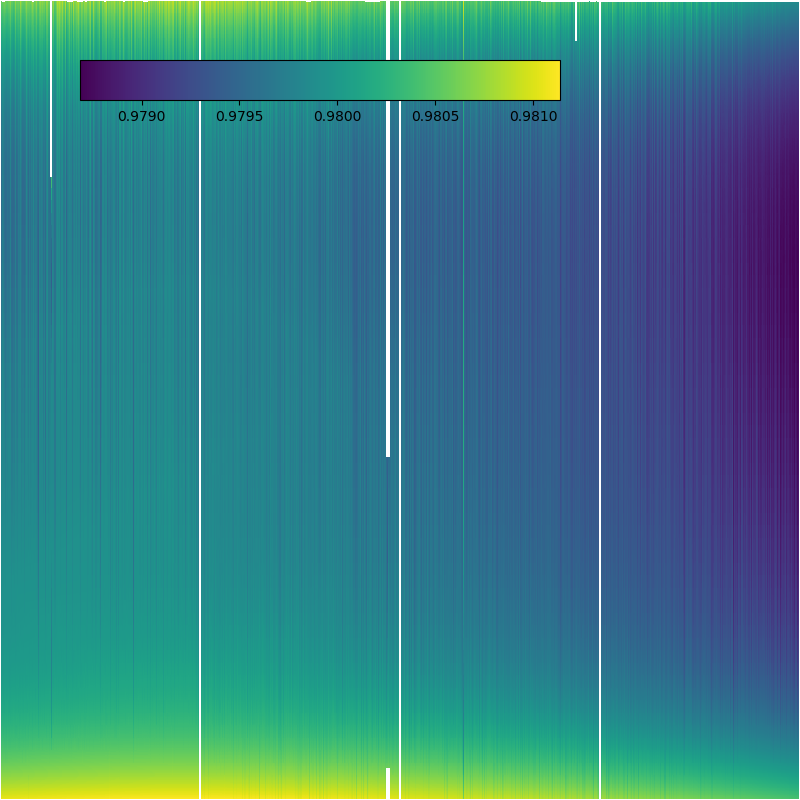Figure 7: Ratio of 0.5 keV to 0.25 keV bin-width instrument maps

The 0.5 keV bin width instrument map has slightly higher pixel values than the 0.25 keV case, but the difference is small (less than one per cent).

Figure 8: Ratio of variable to 0.25 keV bin-width instrument mapsFigure 8: Ratio of variable to 0.25 keV bin-width instrument maps

Unlike Figure 7, the variable bin width map is generally slightly lower than the 0.25 keV version, although the differences are again at the less than one per cent level. The different energy-binning scheme has resulted in a change to the the large-scale pattern, reflecting both the energy and positional dependence of the effective area.

How do the resulting exposure maps differ?

Figure 9 shows the ratio of the exposure maps created using imap.e1.7.fits and imap.weights.fits:

```unix% punlearn mkexpmap
unix% pset mkexpmap asphistf=7.asphist
unix% pset mkexpmap xygrid="3643.1:4723.1:#1080,3853.4:4933.4:#1080"
unix% pset mkexpmap normalize-
unix% mkexpmap instmap=imap.e1.7.fits outf=emap.e1.7.fits mode=h
unix% mkexpmap instmap=imap.weights.fits outf=emap.weights.fits mode=h
unix% dmimgcalc emap.weights.fits,emap.e1.7.fits outf=emap.ratio.fits op=imgout=img1/img2 mode=h
```

Figure 9: Ratio of 0.25 keV bin-width to mono-chromatic exposure mapsFigure 9: Ratio of 0.25 keV bin-width to mono-chromatic exposure maps

The difference in the exposure maps is similar to the corresponding instrument map comparison shown in Figure 6.

An alternative view is the histogram of the pixel values in the various ratio images, as shown in Figure 10:

Figure 10: Histograms of the ratio images[Version: PNG, postscript, PDF]Figure 10: Histograms of the ratio images

The left plot compares the pixel distribution of the instrument- and exposure-map ratio images comparing the weighted result (bin width of 0.25 keV) to the mono-energetic case. The two distributions are very similar.

The right-hand plot shows how the different binning schemes affect the instrument map: in this particular case the choice of binning scheme does not seem to make a large difference on the overall result, but this is a scientific call to be made for each observation.

This figure was created using the following set of commands, making use of routines from Crates, ChIPS, and ciao_contrib.utils.

First we ensure that the ciao_contrib.utils module is loaded:

```chips> from ciao_contrib.utils import *
```

Now we read in the data we want to plot using the Crates routines read_file and get_piximgvals. We then filter the image values to exclude those pixels with values of 0, and use the filtered set of pixels in the call to simple_hist.

```chips> r1 = read_file("ratio1.fits")

chips> i1 = get_piximgvals(r1)
chips> i2 = get_piximgvals(r2)
chips> i3 = get_piximgvals(r3)
chips> ie = get_piximgvals(re)

chips> ix1 = np.where(i1 > 0)
chips> ix2 = np.where(i2 > 0)
chips> ix3 = np.where(i3 > 0)
chips> ixe = np.where(ie > 0)

chips> h1 = simple_hist(i1[ix1], nbins=20)
chips> h2 = simple_hist(i2[ix2], nbins=20)
chips> h3 = simple_hist(i3[ix3], nbins=20)
chips> he = simple_hist(ie[ixe], nbins=20)
```

With the data available now in the h1, h2, h3, and he variables we can now plot up the histograms (normalized to give the fraction of pixels in each bin):

```chips> add_window(6, 4, 'inches')

chips> add_histogram(h1.xlow, h1.xhigh, h1.y * 1.0 / h1.y.sum())
chips> add_histogram(he.xlow, he.xhigh, he.y * 1.0 / he.y.sum())
chips> set_histogram(["line.style", "dot"])
chips> set_plot_ylabel("Fraction of pixels")

chips> split(1,2)

chips> add_histogram(h2.xlow, h2.xhigh, h2.y * 1.0 / h2.y.sum())
chips> set_histogram(["line.color", "red"])
chips> add_histogram(h3.xlow, h3.xhigh, h3.y * 1.0 / h3.y.sum())
chips> set_histogram(["line.color", "green"])

chips> bind_axes("plot2", "ay1", "plot1", "ay1")
chips> set_yaxis(["ticklabel.visible", False])
chips> set_yaxis("by2", ["ticklabel.visible", True])

chips> limits(X_AXIS, 0.9945, 1.0059)
chips> add_label(1.0025, 0.22, "ratio2.fits", ["color", "red"])
chips> add_label(0.999, 0.22, "ratio3.fits", ["color", "green", "halign", 1])

chips> current_plot("plot1")

chips> add_label(0.63, 0.22, "ratio1.fits", ["valign", 0.5])
chips> add_line(0.61, 0.2, 0.628, 0.2, ["style", "dot"])
chips> add_label(0.63, 0.2, "emap.ratio.fits", ["valign", 0.5])

chips> x1 = get_plot("plot1").leftmargin
chips> x2 = 1 - get_plot("plot2").rightmargin
chips> add_label(0.5*(x1+x2), 0.05, "Pixel value", ["size", 14, "coordsys", "FRAME_NORM", "halign", 0.5])
```

The bind_axes call is used to make sure the y axes of the two plots show the same range. Since the two plots share a common axis, we decide to display the tick labels on the right-hand axis of the second plot. Labels and lines are used to add annotations to each plot (setting the valign or halign attributes of a label to 0.5 centers them vertically or horizontally at that coordinate). The last label is added using the frame-normalized (FRAME_NORM) coordinate system to ensure that it is centered between the plots (we use the get_plot routine to get the coordinates of the plot edges).Note

The comparisons above were all made with the on-axis chip; larger differences may be seen between different weighting schemes for ACIS chips that are further off axis, and so are subject to stronger, energy-dependent, vignetting.

The An Introduction to Exposure Maps document provides background reading on why weighted exposure maps are important, and under what conditions they produce meaningful results.

1. If you wish to make several exposure maps for different bands (e.g. 1-2 keV, 2-4 keV, and 4-10 keV), rerun the script for each of these bands. Any time you use the weighting scheme, the sum of the weights should be equal to one. If one (incorrectly) makes a single set of weights with this script and then subdivides them to weight different bands, the weights of each instrument map would not sum to one. Thus, the instrument and exposure maps that are derived would have effective areas that are much too small.

2. To compute an image which gives the integrated flux over the full energy range, it may be best to first compute flux-corrected images in several narrow energy bands (where the ARF is nearly flat) and then sum those fluxed images together. Weighted exposure maps work well for an energy band where the ARF variation isn't very large, but for a full-band 0.5-10 keV image, it may not be a good idea to compute the flux by dividing the counts image by a single number. This is especially true for cases where the source spectrum varies significantly within the image; in that case, there is no general way to compute a single set of weights which will be sensible for every part of the image.

3. The same spectral weights used by mkinstmap can be used with mkpsfmap to produce a spectrally weighted PSF map.

History

 03 Aug 2009 Updated for CIAO 4.1: spectrum.sl has been replaced by the sherpa_contrib.utils module; see the Changes from CIAO 3.4 section; a comparison of weighted to mono-energetic instrument maps has been added. 09 Feb 2010 updated for CIAO 4.2: the "get_imagevals" command has been renamed to get_piximgvals 12 Mar 2010 Added an example of the essential commands to the synopsis section at the start of the thread, for those users who just need reminding of the syntax; updated some of the figures to use ChIPS for display rather than ds9. 19 Jul 2010 the S-Lang syntax has been removed from this thread as it is not supported in CIAO 4.2 Sherpa v2. Contact the CXC Helpdesk if you need assistance transitioning from S-Lang to Python. 13 Jan 2011 reviewed for CIAO 4.3: no changes 21 Nov 2011 updated the module name in the import statement from sherpa_contrib to sherpa_contrib.utils 24 Jan 2012 reviewed for CIAO 4.4: added a Using the make_instmap_weights script section; separated the in-depth analysis into a "step-by-step" section 03 Dec 2012 Review for CIAO 4.5; same spectral weights can be used by mkpsfmap as part of the wavdetect thread. 02 Dec 2013 Review for CIAO 4.6: the pbkfile parameter of mkinstmap is no-longer used - with the obsfile parameter being set to the event file rather than the aspect histogram - and the examples have been updated to use CALDB 4.5.9. 22 Dec 2014 Review for CIAO 4.7; no changes.